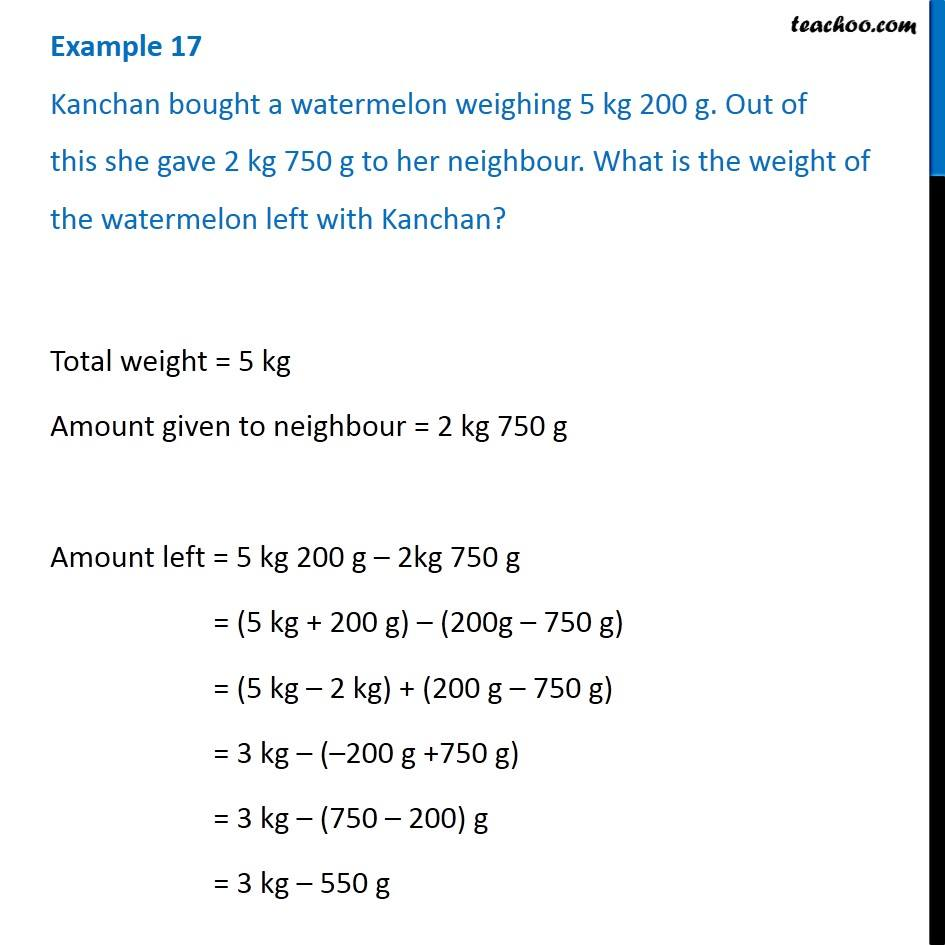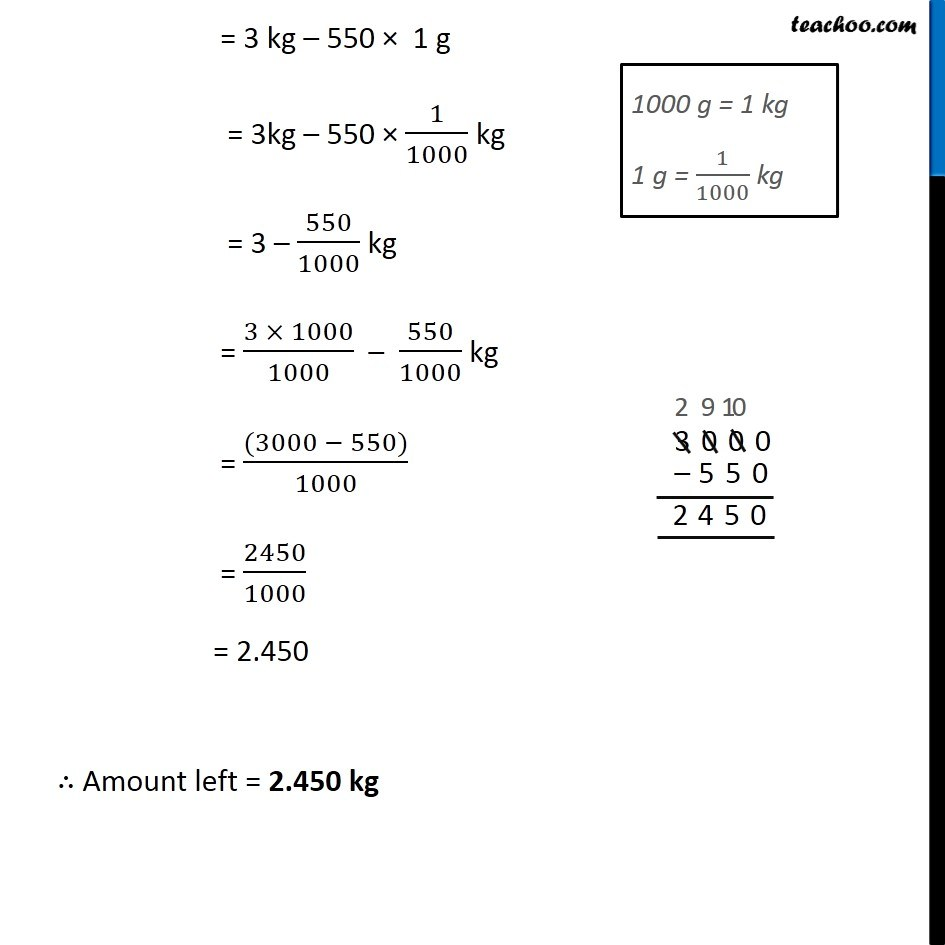Subtraction of decimal numbers - Statement questions

Chapter 8 Class 6 Decimals
Concept wise### Transcript

Example 17 Kanchan bought a watermelon weighing 5 kg 200 g. Out of this she gave 2 kg 750 g to her neighbour. What is the weight of the watermelon left with Kanchan? Total weight = 5 kg Amount given to neighbour = 2 kg 750 g Amount left = 5 kg 200 g – 2kg 750 g = (5 kg + 200 g) – (200g – 750 g) = (5 kg – 2 kg) + (200 g – 750 g) = 3 kg – (–200 g +750 g) = 3 kg – (750 – 200) g = 3 kg – 550 g = 3 kg – 550 × 1 g = 3kg – 550 × 1/1000 kg = 3 – 550/1000 kg = (3 × 1000)/1000 – 550/1000 kg = ((3000 − 550))/1000 = 2450/1000 = 2.450 ∴ Amount left = 2.450 kg